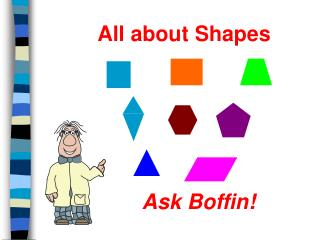DownloadDownload PresentationDownload Presentation- - - - - - - - - - - - - - - - - - - - - - - - - - - E N D - - - - - - - - - - - - - - - - - - - - - - - - - - -
##### Presentation Transcript

2. Properties of Shapes • Number of sides • Number of angles • Number of lines of symmetry • Regular or irregular • Right Angles • Parallel lines

3. Shapes Triangles Quadrilaterals Polygons Regular Shapes Key Words

4. Quadrilaterals Shapes with 4 sides!

5. What shape is this?

6. A Square

7. Properties of a Square • Has 4 equal sides • Has 4 equal angles • Each angle is a right angle • Has 4 lines of symmetry • Is a regular shape

8. What shape is this?

9. A Rectangle

10. Properties of a Rectangle • Has 2 pairs of equal sides • Has 4 equal angles • Each angle is a right angle • Has 2 lines of symmetry • Is an irregular shape

11. What shape is this?

12. A Parallelogram

13. Properties of a Parallelogram • Has 2 pairs of equal sides • Has 2 pairs of equal angles • Opposite sides are parallel • Has NO lines of symmetry • Is an irregular shape

14. What shape is this?

15. A Rhombus

16. Properties of a Rhombus • Has 4 equal sides • Has 2 pairs of equal angles • Opposite sides are parallel • Has 2 lines of symmetry • Is an irregular shape

17. What shape is this?

18. A Trapezium

19. 1 Because these angles are equal. How many lines of symmetry has thisTrapezium got?

20. Properties of a Trapezium • Has unequal sides • One pair of opposite sides are parallel • Usually has NO lines of symmetry • Is an irregular shape

21. What shape is this?

22. A Kite

23. Properties of a Kite • Has 2 pairs of equal sides • Has 1 pair of equal angles • Equal sides are adjacent • Has 1 line of symmetry • Is an irregular shape

24. Quadrilaterals Shapes with 4 sides!

25. Triangles Shapes with 3 sides!

26. What type of triangle is this?

27. An Equilateral Triangle

28. Properties of an Equilateral Triangle • Has 3 equal sides • Has 3 equal angles • Each angle is a 60o angle • Has 3 lines of symmetry • Is a regular shape

29. What type of triangle is this?

30. An Isosceles Triangle

31. Properties of an Isosceles Triangle • Has 2 equal sides • Has 2 equal angles • Has 1 line of symmetry • Is an irregular shape

32. What type of triangle is this?

33. A Scalene Triangle

34. Properties of an Scalene Triangle • Has NO equal sides • Has NO equal angles • Has NO lines of symmetry • Is an irregular shape

35. What type of triangle is this?

36. A Right Angled Triangle

37. A Right Angled Triangle is different It may be scalene or it may be isosceles But it always has a right angle!

38. Properties of a Right Angled Triangle It will be isosceles and have 1 line of symmetry when these 2 sides are equal. • Has 1 right angle • May be an isosceles triangle • May have 1 line of symmetry • Is an irregular shape

39. Triangles Shapes with 3 sides!

40. Key Words • Regular Shape • Parallel • Perpendicular • Vertex • Diagonal • Bisect

41. Regular Shape Shapes are regular when • All their sides are the same length • All their angles are the same size